# Mathematics

### Chapter : Linear Equation In Two Variable

#### Method of Elimination Equation of Coeffieients

Step I : Let the two linear equations obtained be
a1x + b1y + c1 = 0 ....(1)
a2x + b2y + c2 = 0 ....(2)
Step II : Multiplying the given equation so as to make the co-efficients of the variable to be eliminated equal.
Step III : Add or subtract the equations so obtained in Step II, as the terms having the same co-efficients may be either of opposite or the same sign.
Step IV : Solve the equations in one varibale so obtained in Step III.
Step V : Substitute the value found in Step IV in any one of the given equations and then copmpute the value of the other variable.

Solving Simultaneous Linear Equations in one variables

Exampe: Solve the following system of linear equations by applying the method of elimination by equating the co-efficients :
(i) 4x – 3y = 4 and 2x + 4y (ii) 5x – 6y = 8 and 3x + 2y = 6

Solution: (i) We have the linear equations are
4x – 3y = 4 ....(1)
2x + 4y = 3 ....(2)
Let us decide to eliminate x from the given equation. Here, the co-efficients of x are 4 and 2 respectively. We find the L.C.M. of 4 and 2 is 4. Then, make the co-efficients of x equal to 4 in the two equations.
Multiplying equation (1) with 1 and equation (2) with 2, we get ;
4x – 3y = 4 ....(3)
4x + 8y = 6 ....(4)
Subtracting equation (4) from (3), we get ;
–11y = –2
⇒ y =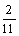Substituting y = 2/11 in equation (1), we get;
4x – 3 ×= 4
⇒ 4x –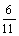= 4
⇒ 4x = 4 +⇒ 4x =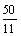⇒ x =Hence, solution of the given system of equation is :
x =, y =(ii) We have;
5x – 6y = 8 ....(1)
3x + 2y = 6 ....(2)
Let us eliminate y from the given system of equations. The co-efficients of y in the given equations are 6 and 2 respectively. The L.C.M. of 6 and 2 is 6. We have to make the both coefficients equal to 6. So, multiplying both sides of equation (1) with 1 and equation (2) with 3, we get ;
5x – 6y = 8 ....(3)
9x + 6y = 18 ....(4)Adding equation (3) and (4), we get ;
14x = 26 ⇒ x =Putting x = 13/7 in equation (1), we get ;– 6y = 8 ⇒– 6y = 8
⇒ 6y =– 8 ==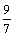⇒ y =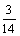Hence, the solution of the system of equations is x =, y =.

Example: Solve the following system of equations by using the method of elimination by equating the co-efficients.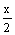++ 2 = 10;+ 1 = 9

Solution: The given system of equation is++ 2 = 10 ⇒+= 8 ...(1) and–+ 1- 9 ⇒–= 8 ....(2)
The equation (1) can be expressed as :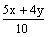= 8 ⇒ 5x + 4y = 80 ....(3)
Similarly, the equation (2) can be expressed as := 8 ⇒ 4x – 7y = 112 ....(4)
Now the new system of equations is
5x + 4y = 80 ....(5)
4x – 7y = 112 ....(6)
Now multiplying equation (5) by 4 and equation (6) by 5, we get ;
20x – 16y = 320 ....(7)
20x + 35y = 560 ....(8)
Subtracting equation (7) from (8), we get ;
y =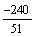Putting y =in equation (5), we get ;
5x + 4 ×= 80 ⇒ 5x –= 80
⇒ 5x = 80 +=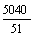⇒ x =⇒ x =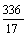Hence, the solution of the system of equations is, x =, y =.

Solve the following system of linear equations by usnig the method of elimination by equating the coefficients : 3x + 4y = 25 ; 5x – 6y = – 9
Sol. The given system of equations is
3x + 4y = 25 ....(1)
5x – 6y = – 9 ....(2)
Let us eliminate y. The coefficients of y are 4 and – 6. The LCM of 4 and 6 is 12. So, we make the coefficients of y as 12 and – 12.
Multiplying equation (1) by 3 and equation (2) by 2, we get
9x + 12y = 75 ....(3)
10x – 12y = – 18 ....(4)
Adding equation (3) and equation (4), we get
19x = 57 ⇒ x = 3.
Putting x = 3 in (1), we get,
3 × 3 + 4y = 25
⇒ 4y = 25 – 9 = 16 ⇒ y = 4
Hence, the solution is x = 3, y = 4.
Verification : Both the equations are satisfied by x = 3 and y = 4, which shows that the solution is correct.

Ex.23 Solve the following system of equations : 15x + 4y = 61; 4x + 15y = 72
Sol. The given system of equation is
15x + 4y = 61 ....(1)
4x + 15y = 72 ....(2)
Let us eliminate y. The coefficients of y are 4 and 15. The L.C.M. of 4 and 15 is 60. So, we make the coefficients of y as 60. Multiplying (1) by 15 and (2) by 4, we get
225x + 60y = 915 ....(3)
16x + 60y = 288 ....(4)
Substracting (4) from (3), we get
209x = 627 ⇒ x =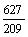= 3
Putting x = 3 in (1), we get
15 × 3 + 4y = 61 ⇒ 45 + 4y = 61
⇒ 4y = 61 – 45 = 16 ⇒ y == 4
Hence, the solution is x = 3, y = 4.
Verification : On putting x = 3 and y = 4 in the given equations, they are satisfied. Hence, the solution is correct.

Ex.26 Solve the following system of linear equations : 2(ax – by) + (a + 4b) = 0 and 2(bx + ay) + (b – 4a) = 0
Sol. 2ax – 2by + a + 4b = 0 .... (1)
2bx + 2ay + b – 4a = 0 .... (2)
Multiplyng (1) by b and (2) by a and subtracting, we get
2(b2 + a2) y = 4 (a2 + b2) ⇒ y = 2
Multiplying (1) by a and (2) by b and adding, we get
2(a2 + b2) x + a2 + b2 = 0
⇒ 2(a2 + b2) x = – (a2 + b2) ⇒ x = –Hence x = –, and y = 2

Ex.27 Solve (a – b) x + (a + b) y = a2 – 2ab – b2

(a + b) (x + y) = a2 + b2
Sol. The given system of equation is
(a – b) x + (a + b) y = a2 – 2ab – b2 ....(1)
(a + b) (x + y) = a2 + b2 ....(2)
 (a + b) x + (a + b) y = a2 + b2 ....(3)
Subtracting equation (3) from equation (1), we get
(a – b) x – (a + b) x = (a2 – 2ab– b2) – (a2 + b2)
 –2bx = – 2ab – 2b2
 x = – = a + b
Putting the value of x in (1), we get
(a – b) (a + b) + (a + b) y = a2 – 2ab – b2
 (a + b) y = a2 – 2ab – b2 – (a2 – b2)
 (a + b) y = – 2ab
y =
 Hence, the solution is x = a + b,
y =